# Difference between revisions of "Prime gap"

## Definition

The prime gap between a primeand its successor primeis the difference. In other words, a prime gap is a gap between two successive primes.

## Facts

We are interested in three broad things:

• How frequently does a given prime gap occur?
• The limit inferior of prime gaps, i.e., as we go to infinity, what is the limiting value of smallest prime gaps?
• The limit superior of prime gaps, i.e., as we go to infinity, what is the limiting value of largest prime gaps?

### Basic facts (lower bound on limit inferior, limit superior is infinity)

• A prime gap ofoccurs betweenand, and never again. All other prime gaps are even, and at least.
• There exist arbitrarily large prime gaps: This is because there exist arbitrarily large sequences of consecutive composite integer. For instance, for any, the sequence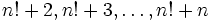is a sequence of composite integers.

### Conjectures and advanced facts on upper bounds on limit inferior and occurrence of small prime gaps

Name of conjecture/fact Statement Status
twin primes conjecture There exist arbitrarily large pairs of twin primes -- successive primes with a gap of two. Equivalently, the limit inferior of prime gaps is exactly 2. open
Polignac's conjecture For any natural number, the prime gapoccurs for arbitrarily large pairs of primes open; stronger than twin primes conjecture
Goldston-Pintz-Yildirim theorem on prime gaps conditional to Elliott-Halberstam there exist infinitely many pairs of consecutive primes with prime gap at most 16 proof conditional to Elliott-Halberstam conjecture
Zhang's theorem on bounded prime gaps There exist infinitely many pairs of primes that differ by at most 70 million. In other words, there eixsts a prime gapthat is at most 70 million that occurs infinitely often. Subsequent work by the Polymath project brought the bound down to 4680 Closed, see here
Maynard's theorem on bounded prime gaps There exist infinitely many pairs of primes that differ by at most 600. Closed, see here

### Conjectures and advanced facts on maximum prime gaps (growth of limit superior relative to size of numbers)

Name of conjecture/fact Statement Function (big-O) Status
Cramér's prime gap conjecture For any prime, the prime gap betweenand the next prime is at most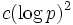,fixedopen
Prime-between-squares conjecture There exists a prime between any two successive squares. Puts upper bound of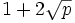on prime gapopen
(corollary of) Generalized Riemann hypothesis The prime gap between a primeand the next prime is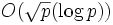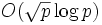open
exponent bound for prime gap of 0.535 The prime gap betweenand the next prime is at most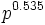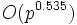proved
(corollary of) prime number theorem there exists a prime betweenand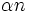for any, forlarge enough (dependent on)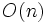proved
Bertrand's postulate there exists a prime betweenandproved